# Standard #: MA.3.FR.2.1

This document was generated on CPALMS - www.cpalms.org

Plot, order and compare fractional numbers with the same numerator or the same denominator.

### Examples

The fraction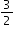is to the right of the fraction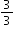on a number line sois greater than.

### Clarifications

Clarification 1: Instruction includes making connections between using a ruler and plotting and ordering fractions on a number line.
Clarification 2: When comparing fractions, instruction includes an appropriately scaled number line and using reasoning about their size.

Clarification 3: Fractions include fractions greater than one, including mixed numbers, with denominators limited to 2, 3, 4, 5, 6, 8, 10 and 12.

### General Information

Subject Area: Mathematics (B.E.S.T.)
Strand: Fractions
Status: State Board Approved

• Number Line

### Vertical Alignment

Previous Benchmarks

Next Benchmarks

### Purpose and Instructional Strategies

The purpose of this benchmark is for students to plot and order fractions with the same numerator (e.g., $\frac{\text{3}}{\text{4}}$, $\frac{\text{3}}{\text{2}}$, $\frac{\text{3}}{\text{8}}$) or fractions with the same denominator (e.g., $\frac{\text{3}}{\text{5}}$, $\frac{\text{10}}{\text{5}}$, $\frac{\text{7}}{\text{5}}$) to compare them by their location on a number line.
• During instruction, teachers should provide students opportunities to practice using the number line, which will assist students with understanding the difference in size when fractions have the same numerator (the size of the parts) and with comparing fractions with the same denominator (number of parts) (MTR.2.1).
• Through making connections to rulers, students see that appropriately scaled number lines allow for comparisons of fraction size. Students should also utilize open number lines as to practice creating their own appropriately scaled number lines (MTR.2.1).
• Instruction should model that fractional units on a number line represent intervals that are its unit fraction in size. For example, $\frac{\text{5}}{\text{3}}$ on a number line is represented by 5 units from 0 that are each one-third in length. Second, number lines help students see comparisons of fractions to the same whole and will continue as students compare fractions with different numerators and denominators in Grade 4. Finally, number lines reinforce Clarification 3 for MA.3.FR.1.3, that fractions are numbers (MTR.2.1, MTR.5.1).

### Common Misconceptions or Errors

• Students can be confused that when numerators are the same in fractions, larger denominators represent smaller pieces, and smaller denominators represent larger pieces.
• When fraction comparisons are made using area models, students may be confused that the size of the whole for each model must be the same size.

### Strategies to Support Tiered Instruction

• Instruction includes opportunities to use concrete models and drawing of number lines to connect learning with fraction understanding.
• For example, students plot fourths on the number line. Utilizing fraction strips or tiles, students can connect fractional parts to the measurement on a number line.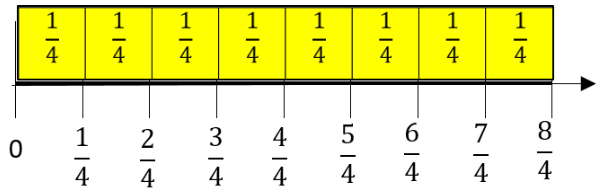• Conversation includes what students notice about the fraction on the number line. “How many fourths are in three-fourths? What do we notice about the size of $\frac{\text{1}}{\text{4}}$ compared to $\frac{\text{3}}{\text{4}}$?” Students have opportunities to describe the distance from the 0 as well as the distance from other benchmark fractions.
• Instruction includes opportunities to use fraction manipulatives, concrete models, and drawings. The teacher begins instruction by modeling fractional pieces with their fraction name. It is important that students see that the fractions that they are building and comparing refer to the same size whole.
• For example, students build fractions tiles or models to equal the same size one whole like below.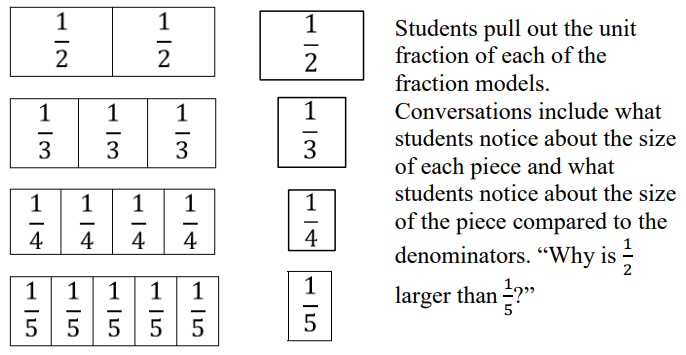Clara says that $\frac{\text{5}}{\text{4}}$ is greater than $\frac{\text{5}}{\text{2}}$ because 4 is greater than 2. Prove why she is incorrect using the number line below.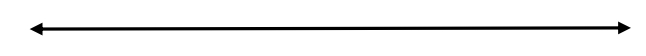### Instructional Items

Instructional Item 1

Order the fractions below from least to greatest.
$\frac{\text{8}}{\text{5}}$, $\frac{\text{8}}{\text{3}}$, $\frac{\text{8}}{\text{10}}$, $\frac{\text{8}}{\text{1}}$

Instructional Item 2

Compare 7 fourths and 3 fourths using <, =, or >.

*The strategies, tasks and items included in the B1G-M are examples and should not be considered comprehensive.

#### Related Courses

 Course Number1111 Course Title222 5012050: Grade Three Mathematics (Specifically in versions: 2014 - 2015, 2015 - 2022, 2022 and beyond (current)) 7712040: Access Mathematics Grade 3 (Specifically in versions: 2014 - 2015, 2015 - 2018, 2018 - 2022, 2022 and beyond (current)) 5012055: Grade 3 Accelerated Mathematics (Specifically in versions: 2019 - 2022, 2022 and beyond (current)) 5012015: Foundational Skills in Mathematics 3-5 (Specifically in versions: 2019 - 2022, 2022 and beyond (current))

#### Related Access Points

 Access Point Number Access Point Title MA.3.FR.2.AP.1 Compare fractional numbers with the same denominator. Denominators are limited to 2, 3 and 4.

#### Formative Assessments

 Name Description Comparing Fractions Students compare two pairs of fractions and record their comparisons using the less than or greater than symbols. The Cake Problem Students compare two fractional parts of two different wholes. Four-Sixths on the Number Line Students are asked to use a number line that includes the location of zero and one-sixth to find the location of four-sixths. One-Third on the Number Line Students are given four number line diagrams and asked to choose the one that correctly shows the location of one-third. Five-Eighths on the Number Line Students are asked to locate five-eighths on a number line that has been anchored by zero and one, but that has not yet been scaled. Three-Fourths on the Number Line Students are asked to scale a number line from zero to one so that they can find the location of three-fourths.

#### Image/Photograph

 Name Description Clipart ETC Fractions Illustrations that can be used for teaching and demonstrating fractions. Fractional representations are modeled in wedges of circles ("pieces of pie") and parts of polygons. There are also clipart images of numerical fractions, both proper and improper, from halves to twelfths. Fraction charts and fraction strips found in this collection can be used as manipulatives and are ready to print for classroom use.

#### Original Student Tutorials

 Name Description Fraction Camp! Fractions Greater Than 1 on a Number Line Joey uses his knowledge of fractions to win games at camp by knowing where fractions greater than one are located on number lines, in this interactive tutorial. Nature Walk: Fractions Less Than 1 on a Number Line Learn to use number lines to represent fractions as Emmy explores nature in this interactive tutorial. Finding Fractions at Camp: Fractions on a Number Line Joey learns about the location of unit fractions on a number line while at camp in this interactive tutorial.

#### Original Student Tutorials

 Name Description Fraction Camp! Fractions Greater Than 1 on a Number Line: Joey uses his knowledge of fractions to win games at camp by knowing where fractions greater than one are located on number lines, in this interactive tutorial. Nature Walk: Fractions Less Than 1 on a Number Line: Learn to use number lines to represent fractions as Emmy explores nature in this interactive tutorial. Finding Fractions at Camp: Fractions on a Number Line: Joey learns about the location of unit fractions on a number line while at camp in this interactive tutorial.

#### Image/Photograph

 Name Description Clipart ETC Fractions: Illustrations that can be used for teaching and demonstrating fractions. Fractional representations are modeled in wedges of circles ("pieces of pie") and parts of polygons. There are also clipart images of numerical fractions, both proper and improper, from halves to twelfths. Fraction charts and fraction strips found in this collection can be used as manipulatives and are ready to print for classroom use.

Printed On:9/25/2023 3:10:54 AM
Print Page | Close this window